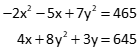## Self Study Problems

### Problem (1)

Determine the values for a, b, and c to 0.1 for the following equations.

2a - 4b + 3c = 14.5
-a + 2b + c = -6.0
5a - b - 4c = 8.0

### Problem (2)

A +4.0% grade is followed by a -2.0% grade on a vertical alignment. Using the information shown below, what are the station and elevation of the PVI?Use a Taylor Series approximation to compute the variable values in Problems 3 and 4. Determine to 0.1.

### Problem (3)

0.5x3 - 4x2 + 2.5x = -79.2

### Problem (4)